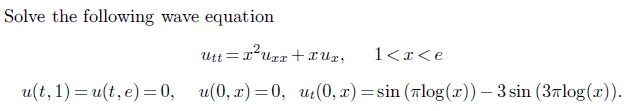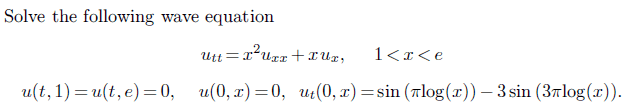# Solving a Wave Equation

• Precursor

#### Precursor

Homework StatementThe attempt at a solution

I'm using the method of separation of variables by first defining the solution as $u(x,t) =X(x)T(t)$

Putting this back into the PDE I get: $T''X = x^{2}X''T + xX'T$

which is simplified to $$\frac{T''}{T} = \frac{x^{2}X'' + xX'}{X} = -\lambda^{2}$$

The spatial problem is then: $x^{2}X'' + xX' = -X\lambda^{2}$

Is this correct so far? How do I continue?

Yes, that is correct. You proceed, of course, by solving that equation, together with the boundary conditions X(1)= 0, X(e)= 0.

(Those boundary conditions follow from u(t, 1)= T(t)X(1)= 0 and u(t, e)= T(t)X(e)= 0. If T(t) is not identically 0, which would not satisfy the initial conditions, then X(1)= X(e)= 0.)

(An obvious solution is the trivial X(x)= 0. But then you could not satisfy the initial conditions. $\lambda$ must be such that the equation has non-trivial solutions- i.e. eigenvalues.)

Homework StatementThe attempt at a solution

I'm using the method of separation of variables by first defining the solution as $u(x,t) =X(x)T(t)$

Putting this back into the PDE I get: $T''X = x^{2}X''T + xX'T$

which is simplified to $$\frac{T''}{T} = \frac{x^{2}X'' + xX'}{X} = -\lambda^{2}$$

The spatial problem is then: $x^{2}X'' + xX' = -X\lambda^{2}$

Is this correct so far? How do I continue?

Looking at the boundary conditions, the substitution $X(x) = Z(\log (x))$ looks helpful.